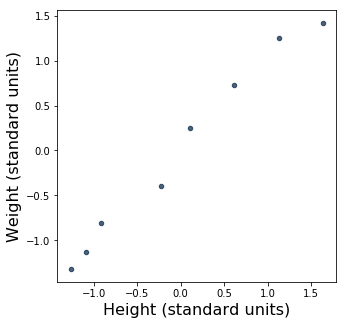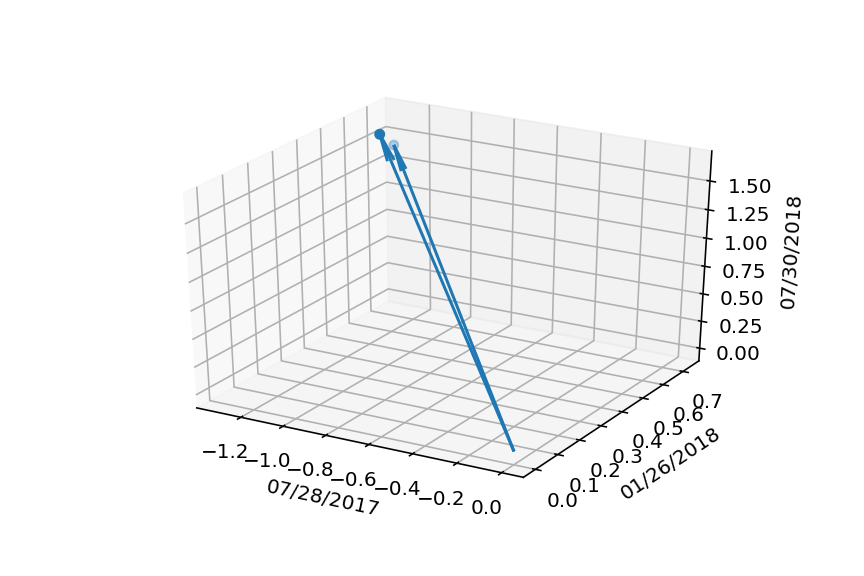# Way number eight of looking at the correlation coefficient¶

This is a notebook to accompany the blog post "Way number eight of looking at the correlation coefficient". Read the post for additional context!

In :
import numpy as np
from datascience import *
from datetime import *
import matplotlib
%matplotlib inline
import matplotlib.pyplot as plots
from mpl_toolkits.mplot3d import Axes3D
import pandas as pd
import math


## Recap from last time¶

As before, we're using the datascience package, and everything else we're using is pretty standard.

And, as before, here's the data we'll be working with, converted to standard units and plotted:

In :
heightweight = Table().with_columns([
'Date',        ['07/28/2017', '08/07/2017', '08/25/2017', '09/25/2017', '11/28/2017', '01/26/2018', '04/27/2018', '07/30/2018'],
'Height (cm)', [        53.3,         54.6,         55.9,           61,         63.5,         67.3,         71.1,         74.9],
'Weight (kg)', [       4.204,         4.65,        5.425,         6.41,        7.985,        9.125,        10.39,       10.785],
])
def standard_units(nums):
return (nums - np.mean(nums)) / np.std(nums)

heightweight_standard = Table().with_columns(
'Date', heightweight.column('Date'),
'Height (standard units)', standard_units(heightweight.column('Height (cm)')),
'Weight (standard units)', standard_units(heightweight.column('Weight (kg)')))
heightweight_standard

Out:
Date Height (standard units) Weight (standard units)
07/28/2017 -1.26135 -1.3158
08/07/2017 -1.08691 -1.13054
08/25/2017 -0.912464 -0.808628
09/25/2017 -0.228116 -0.399485
11/28/2017 0.107349 0.254728
01/26/2018 0.617255 0.728253
04/27/2018 1.12716 1.2537
07/30/2018 1.63707 1.41777
In :
heightweight_standard.scatter(
'Height (standard units)',
'Weight (standard units)')## Visualizing the data in "person space"¶

So far, this is all a recap of last time. Now, let's try turning our data sideways.

The hacky way I have of doing this is to convert the data first to a numpy ndarray, then to a pandas DataFrame, and then transposing the DataFrame. This is kind of silly, but I don't know a better way to transpose a structured ndarray. If you do, let me know.

In :
# First convert to a plain old numpy ndarray.
heightweight_standard_np = heightweight_standard.to_array()

# Now convert *that* to a pandas DataFrame.
df = pd.DataFrame(heightweight_standard_np)

# Get the transpose of the DataFrame.
df = df.T
df

Out:
0 1 2 3 4 5 6 7
Date 07/28/2017 08/07/2017 08/25/2017 09/25/2017 11/28/2017 01/26/2018 04/27/2018 07/30/2018
Height (standard units) -1.26135 -1.08691 -0.912464 -0.228116 0.107349 0.617255 1.12716 1.63707
Weight (standard units) -1.3158 -1.13054 -0.808628 -0.399485 0.254728 0.728253 1.2537 1.41777

pandas defaults to using RangeIndex (0, 1, 2, …, n) for the column labels, but we want the dates from the first row to be the column headers rather than being an actual row. That's an easy change to make, though.

In :
df.columns = df.iloc
df = df.drop("Date")
df

Out:
Date 07/28/2017 08/07/2017 08/25/2017 09/25/2017 11/28/2017 01/26/2018 04/27/2018 07/30/2018
Height (standard units) -1.26135 -1.08691 -0.912464 -0.228116 0.107349 0.617255 1.12716 1.63707
Weight (standard units) -1.3158 -1.13054 -0.808628 -0.399485 0.254728 0.728253 1.2537 1.41777

While we're at it, we'll convert the values in our DataFrame to numeric values, so that we can visualize them in a moment.

In :
df = df.apply(pd.to_numeric)
df

Out:
Date 07/28/2017 08/07/2017 08/25/2017 09/25/2017 11/28/2017 01/26/2018 04/27/2018 07/30/2018
Height (standard units) -1.261347 -1.086906 -0.912464 -0.228116 0.107349 0.617255 1.127161 1.637068
Weight (standard units) -1.315798 -1.130542 -0.808628 -0.399485 0.254728 0.728253 1.253700 1.417773

Eight dimensions are too many to try to visualize, but we can pare it down to three. We'll pick three -- the first (07/28/2017), the last (07/30/2018), and one in the middle (01/26/2018) -- and drop the rest.

In :
df_3dim = df.drop(df.columns[[1, 2, 3, 4, 6]],axis=1)
df_3dim

Out:
Date 07/28/2017 01/26/2018 07/30/2018
Height (standard units) -1.261347 0.617255 1.637068
Weight (standard units) -1.315798 0.728253 1.417773

Now we can visualize the data with a three-dimensional scatter plot.

In :
%matplotlib notebook
scatter_3d = plots.figure().gca(projection='3d')
scatter_3d.scatter(df_3dim.iloc[:, 0], df_3dim.iloc[:, 1], df_3dim.iloc[:, 2])
scatter_3d.set_xlabel(df_3dim.columns)
scatter_3d.set_ylabel(df_3dim.columns)
scatter_3d.set_zlabel(df_3dim.columns)
height_point = df_3dim.iloc
weight_point = df_3dim.iloc
origin = [0,0,0]

X, Y, Z = zip(origin,origin)
U, V, W = zip(height_point, weight_point)

scatter_3d.quiver(X, Y, Z, U, V, W, arrow_length_ratio=0.09)

plots.show()What's going on here? We're in the "person space", where, as Rodgers and Nicewander explained, each axis represents an observation -- in this case, three observations. And there are two points, as promised -- one for each of height and weight.

If we look at the difference between the two points on the z-axis -- that is, the axis for 07/30/2018 -- the darker-colored blue dot is higher up, so it must represent the height variable, with coordinates (-1.26135, 0.617255, 1.63707) That means that the other, lighter-colored blue dot, with coordinates (-1.3158, 0.728253, 1.41777), must represent the weight variable.

I've also plotted vectors going from the origin to each point. These are the "variable vectors" for the two points.

## The angle between the variable vectors¶

Finally, we want to figure out the angle between the two vectors. There are various ways to do that in Python; we'll use a simple one that works for us:

In :
def dotproduct(v1, v2):
return sum((a*b) for a, b in zip(v1, v2))

def length(v):
return math.sqrt(dotproduct(v, v))

def angle(v1, v2):
return math.acos(dotproduct(v1, v2) / (length(v1) * length(v2)))

angle_between_vvs = angle(height_point, weight_point)
angle_between_vvs

Out:
0.11140728370937446

Finally, we can take the cosine of that to get the correlation coefficient $r$:

In :
math.cos(angle_between_vvs)

Out:
0.9938006245545371

Almost 1! That means that, just like last time, we have an almost perfect linear correlation.

It's a bit different from what we had last time, though, which was 0.9910523777994954. That's because, for the sake of visualization, we decided to only look at three of the observations.

## The angle between the actual variable vectors¶

We can, however, go back to all eight dimensions. We may not be able to visualize them, but we can still measure the angle between them!

In :
height_point_8dim = df.iloc
weight_point_8dim = df.iloc
angle_between_8dim_vvs = angle(height_point_8dim, weight_point_8dim)
angle_between_8dim_vvs

Out:
0.1338730551963976

Taking the cosine of this slightly bigger angle:

In :
math.cos(angle_between_8dim_vvs)

Out:
0.9910523777994951

This turns out to be the same as what we had previously calculated $r$ to be, modulo a little numerical imprecision. And so, that's way number eight of looking at the correlation coefficient -- as the angle between two variable vectors in "person space".